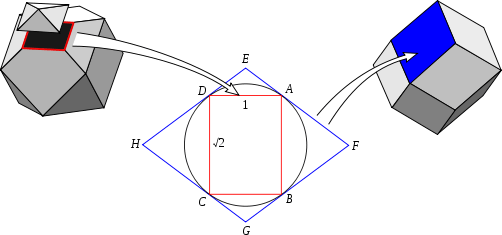# Dual polygon

In geometry, polygons are associated into pairs called duals, where the vertices of one correspond to the edges of the other.

## PropertiesDorman Luke construction, showing a rhombus face being dual to a rectangle vertex figure.

The dual of an isogonal (vertex-transitive) polygon is an isotoxal (edge-transitive) polygon. For example, the (isogonal) rectangle and (isotoxal) rhombus are duals.

In a cyclic polygon, longer sides correspond to larger exterior angles in the dual (a tangential polygon), and shorter sides to smaller angles. Further, congruent sides in the original polygon yields congruent angles in the dual, and conversely. For example, the dual of a highly acute isosceles triangle is an obtuse isosceles triangle.

In the Dorman Luke construction, each face of a dual polyhedron is the dual polygon of the corresponding vertex figure.

As an example of the side-angle duality of polygons we compare properties of the cyclic and tangential quadrilaterals.

Circumscribed circle Inscribed circle
Perpendicular bisectors of the sides are concurrent at the circumcenter Angle bisectors are concurrent at the incenter
The sums of the two pairs of opposite angles are equal The sums of the two pairs of opposite sides are equal

This duality is perhaps even more clear when comparing an isosceles trapezoid to a kite.

Isosceles trapezoid Kite
Two pairs of equal adjacent angles Two pairs of equal adjacent sides
One pair of equal opposite sides One pair of equal opposite angles
An axis of symmetry through one pair of opposite sides An axis of symmetry through one pair of opposite angles
Circumscribed circle Inscribed circle

## Kinds of duality

### Rectification

The simplest qualitative construction of a dual polygon is a rectification operation, where the edges of a polygon are truncated down to vertices at the center of each original edge. New edges are formed between these new vertices.

This construction is not reversible. That is, the polygon generated by applying it twice is in general not similar to the original polygon.

### Polar reciprocation

As with dual polyhedra, one can take a circle (be it the inscribed circle, circumscribed circle, or if both exist, their midcircle) and perform polar reciprocation in it.

### Projective duality

Under projective duality, the dual of a point is a line, and of a line is a point – thus the dual of a polygon is a polygon, with edges of the original corresponding to vertices of the dual and conversely.

From the point of view of the dual curve, where to each point on a curve one associates the point dual to its tangent line at that point, the projective dual can be interpreted thus:

• every point on a side of a polygon has the same tangent line, which agrees with the side itself – they thus all map to the same vertex in the dual polygon
• at a vertex, the "tangent lines" to that vertex are all lines through that point with angle between the two edges – the dual points to these lines are then the edge in the dual polygon.

### Combinatorially

Combinatorially, one can define a polygon as a set of vertices, a set of edges, and an incidence relation (which vertices and edges touch): two adjacent vertices determine an edge, and dually, two adjacent edges determine a vertex. Then the dual polygon is obtained by simply switching the vertices and edges.

Thus for the triangle with vertices {A,B,C} and edges {AB,BC,CA}, the dual triangle has vertices {AB,BC,CA}, and edges {B,C,A}, where B connects AB & BC, and so forth.

This is not a particularly fruitful avenue, as combinatorially, there is a single family of polygons (given by number of sides); geometric duality of polygons is more varied, as are combinatorial dual polyhedra.